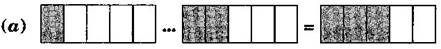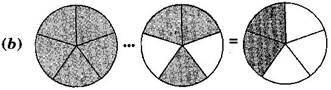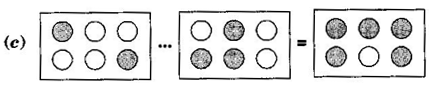### Fractions-Solutions Ex-7.5

CBSE Class –VI Mathematics
NCERT Solutions
Chapter 7 Fraction (Ex. 7.5)

Question 1. Write the fractions appropriately as additions or subtractionsAnswer:  (a) $\frac{1}{5}+\frac{2}{5}=\frac{1+2}{5}=\frac{3}{5}$
(b) $\frac{5}{5}-\frac{3}{5}=\frac{5-3}{5}=\frac{2}{5}$
(c) $\frac{2}{6}+\frac{3}{6}=\frac{2+3}{6}=\frac{5}{6}$
Question 2. Solve:
(a) $\frac{1}{18}+\frac{1}{18}$
(b) $\frac{8}{15}+\frac{3}{15}$
(c) $\frac{7}{7}-\frac{5}{7}$
(d) $\frac{1}{22}+\frac{21}{22}$
(e) $\frac{12}{15}-\frac{7}{15}$
(f) $\frac{5}{8}+\frac{3}{8}$
(g) $1-\frac{2}{3}\left(1=\frac{3}{3}\right)$
(h) $\frac{1}{4}+\frac{0}{4}$
(i) $3-\frac{12}{5}$
Answer: (a) $\frac{1}{18}+\frac{1}{18}=\frac{1+1}{18}=\frac{2}{18}=\frac{1}{9}$
(b) $\frac{8}{15}+\frac{3}{15}=\frac{8+3}{15}=\frac{11}{15}$
(c) $\frac{7}{7}-\frac{5}{7}=\frac{7-5}{7}=\frac{2}{7}$
(d) $\frac{1}{22}+\frac{21}{22}=\frac{1+21}{22}=\frac{22}{22}=1$
(e) $\frac{12}{15}-\frac{7}{15}=\frac{12-7}{15}=\frac{5}{15}=\frac{1}{3}$
(f) $\frac{5}{8}+\frac{3}{8}=\frac{8}{8}=1$
(g) $1-\frac{2}{3}=\frac{3}{3}-\frac{2}{3}=\frac{3-2}{2}=\frac{1}{3}$
(h) $\frac{1}{4}+\frac{0}{4}=\frac{1+0}{4}=\frac{1}{4}$
(i) $3-\frac{12}{5}=\frac{15}{5}-\frac{12}{5}=\frac{15-12}{5}=\frac{3}{5}$
Question 3. Shubham painted $\frac{2}{3}$ of the wall space in his room. His sister Madhavi helped and painted $\frac{1}{3}$ of the wall space. How much did they paint together?
Answer: Fraction of the wall painted by Shubham = $\frac{2}{3}$
Fraction of the wall painted by Madhavi = $\frac{1}{3}$
Total painting done by both of them = $\frac{2}{3}+\frac{1}{3}=\frac{2+1}{3}=\frac{3}{3}=1$
Therefore, they painted the wall completely.
Question 4. Fill in the missing fractions:
(a)
(b)
(c)
(d)
Answer: (a) $\frac{4}{10}$
(b) $\frac{8}{21}$
(c) $\frac{6}{6}$
(d) $\frac{7}{27}$
Question 5.Javed was given a basket of  7 oranges, He sold only 5 oranges. What fraction of oranges was left in the basket?
Fraction of Orange left = $1-\frac{5}{7}$
$\frac{7}{7}-\frac{5}{7}=\frac{7-5}{7}=\frac{2}{7}$
Thus, $\frac{2}{7}$ oranges was left in the basket.